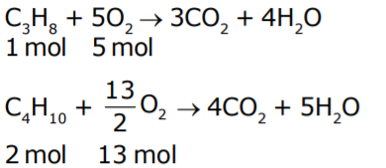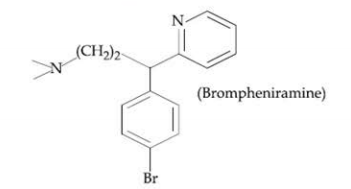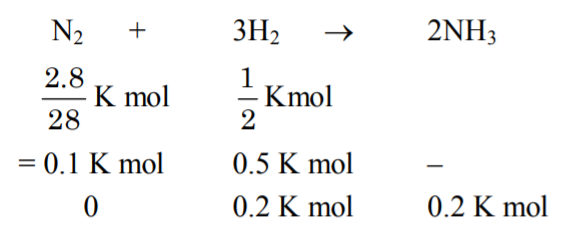Filter By

## All Questions

#### The most abundant elements by mass in the body of a healthy human adult are : Oxygen (61.4%); Carbon (22.9%), Hydrogen (10.0%); and Nitrogen (2.6%). The weight (in kg) which a 75 kg person would gain if all  atoms are replaced by atoms is :   Option: 1 7.5 Option: 2 10 Option: 3 15 Option: 4 37.5

Given that

Mass of the person = 75 kg

Mass of 1H1 present in person = 10% of 75 kg = 7.5 kg

Since Mass of 1H2 is double the Mass of 1H1

So, Mass of 1H2 will be in person = 2 X 7.5 kg =15 kg

Thus, increase in weight = 15 - 7.5 = 7.5 kg

Therefore, Option (1) is correct

#### The correct order of the atomic radii of C, Cs, Al and S is : Option: 1Option: 2Option: 3Option: 4In a period from left to right the effective nuclear charge increases because the next electron fills in the same shell. So the atomic size decrease.

- whereinThe attraction between the outer electrons and the nucleus increases as the atomic radius decreases in a period.

- whereinSize of atom and ion in a group -

In a group moving from top to the bottom the number of shell increases.So the atomic size increases.

- whereinAs we know that

From Left to right in a period size decreases and when going down the group size increasesTherefore, Option(2) is correct

## Crack CUET with india's "Best Teachers"

• HD Video Lectures
• Unlimited Mock Tests
• Faculty Support#### The IUPAC symbol for the element with atomic number 119 would be: Option: 1 uue Option: 2une Option: 3 unh Option: 4 uun

Nomenclature of elements with atomic number >100 -

The name is derived directly from the atomic number of the element using the following numerical roots:

0 = nil

1 = un

2 = bi

3 = tri

5 = pent

6 = hex

7 = sept

8 = oct

9 = enn

Eg:

 Atomic number Name Symbol 101 Mendelevium (Unnilunium) Md (Unu) 102 Nobelium (Unnilbium) No (Unb)

-

uue

1  1  9

Un Un ennium

Therefore, Option(1) is correct.

#### The ammonia  released on quantitative reaction of 0.6g, urea  with sodium hydroxide  can be neutralised by: Option: 1 200 ml of 0.2 N HCl Option: 2200 ml of 0.4 N HCl Option: 3100 ml of 0.1N HCl Option: 4100 ml of 0.2N HCl

2 × mole of Urea = mole of  ........(1)
mole of  = mole of  ........(2)
mole of HCl = 2 × mole of Urea

mole of HCl = ...(i)

[We know , mole = M X V = N X n X V]

...as (i).

Therefore, Option(4) is correct.

## Crack NEET with "AI Coach"

• HD Video Lectures
• Unlimited Mock Tests
• Faculty Support#### The average molar mass of chlorine is The ratio of in naturally occurring chlorine is close to :  Option: 1 4:1Option: 2 3:1Option: 3 2:1Option: 4 1:1

Given,

The average molar mass of chlorine is

Let , the ratio of in naturally occurring chlorine is close to x : y

Now, we know

So,

Therefore, the correct option is (2).

#### The minimum number of moles of O2 required for complete combustion of 1 mole of propane and 2 moles of butane is ______

Combustion reaction of 1 mole of propane and 2 moles of butane-So, Total required mol of O2 = 5 + 13 = 18.

Ans = 18

## Crack JEE Main with "AI Coach"

• HD Video Lectures
• Unlimited Mock Tests
• Faculty Support#### If a person is suffering from the deficiency if nor-adrenaline, what kind of drug can be suggested ?  Option: 1 Anti-inflammatoryOption: 2 AntidepressantOption: 3 AntihistamineOption: 4 Analgesic

Non-adrenaline is a neurotransmitter. If nor-adrenaline is low, a person may suffer from depression. Hence, an antidepressant drug is suggested.

Anti-depressant → drug, which enhances the mood.

Therefore, the correct option is (2).

#### The following molecules act as an :Option: 1 AntidepranantOption: 2 Anti-bacterial  Option: 3 AntisepticOption: 4 Anti-histamine

Brompheniramine works by blocking the action of histamine, which causes allergic symptoms.

Hence, it acts as an antihistamine.

Therefore, Option(3) is correct.

## Crack CUET with india's "Best Teachers"

• HD Video Lectures
• Unlimited Mock Tests
• Faculty Support#### The mass of ammonia in grams produced when 2.8 kg of dinitrogen quantitatively reacts with 1 kg of dihydrogen is_________Option: 1 3400Option: 2 -Option: 3 -Option: 4 -

Given,

2.8 kg of dinitrogen quantitatively reacts with 1 kg of dihydrogen.From above, the mole of ammonia = 0.2 kMol

So, mass of ammonia = mole X molar mass

mass of ammonia = 0.2 (kMol ) X 17 (g/Mol)

mass of ammonia = 3.4 kg = 3400 g

Ans = 3400

#### A 100mL solution was made by adding 1.43g of. The normality of the solution is 0.1N. The value of x is___________. (The atomic mass of Na is 23 g/mol)

Molar mass(M) of Na2CO3.xH2O
M =  23 × 2 + 12 + 48 + 18x
M =  46 + 12 + 48 + 18x
M  =  (106 + 18x)

As nfactor in dissolution will be determined from net cationic or anionic charge; which is 2 so.

Eq.wt. =  = (53 + 9x)

As volume = 100 ml = 0.1 Litre

53 + 9x = 143
9x = 90
x = 10.00

Ans = 10